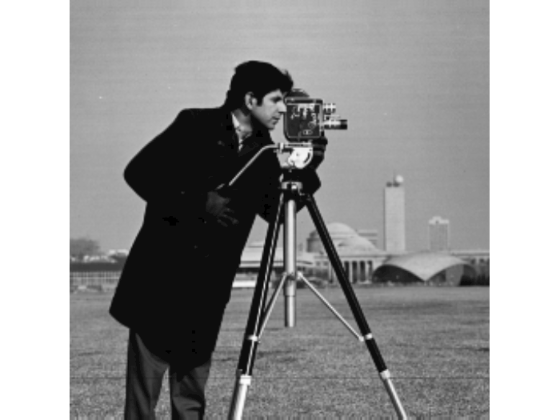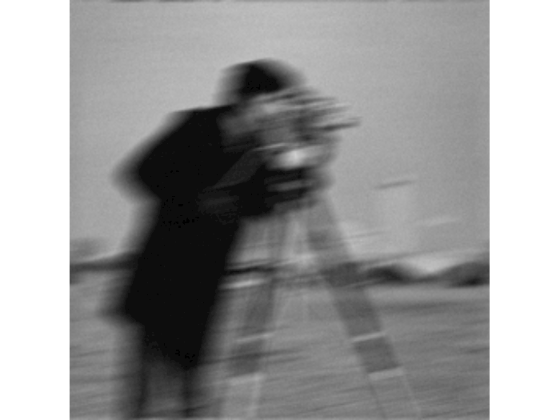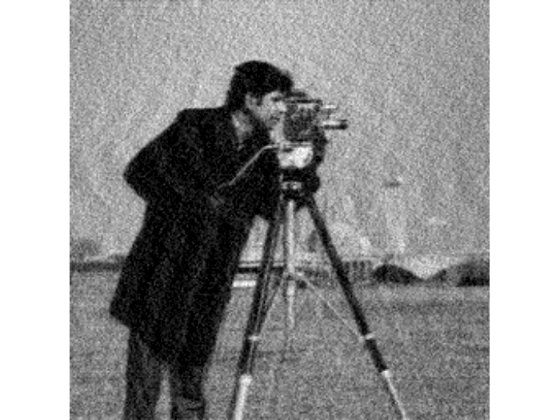Documentation

# deconvwnr

Deblur image using Wiener filter

## Syntax

``J = deconvwnr(I,psf,nsr)``
``J = deconvwnr(I,psf,ncorr,icorr)``

## Description

example

````J = deconvwnr(I,psf,nsr)` deconvolves image `I` using the Wiener filter algorithm, returning deblurred image `J`. `psf` is the point-spread function (PSF) with which `I` was convolved. `nsr` is the noise-to-signal power ratio of the additive noise. The algorithm is optimal in a sense of least mean square error between the estimated and the true images.```
````J = deconvwnr(I,psf,ncorr,icorr)` deconvolves image `I`, where `ncorr` is the autocorrelation function of the noise and `icorr` is the autocorrelation function of the original image. ```

## Examples

collapse all

Read image into the workspace and display it.

```I = im2double(imread('cameraman.tif')); imshow(I); title('Original Image (courtesy of MIT)');```Simulate a motion blur.

```LEN = 21; THETA = 11; PSF = fspecial('motion', LEN, THETA); blurred = imfilter(I, PSF, 'conv', 'circular'); figure, imshow(blurred)```Simulate additive noise.

```noise_mean = 0; noise_var = 0.0001; blurred_noisy = imnoise(blurred, 'gaussian', ... noise_mean, noise_var); figure, imshow(blurred_noisy) title('Simulate Blur and Noise')```Try restoration assuming no noise.

```estimated_nsr = 0; wnr2 = deconvwnr(blurred_noisy, PSF, estimated_nsr); figure, imshow(wnr2) title('Restoration of Blurred, Noisy Image Using NSR = 0')```Try restoration using a better estimate of the noise-to-signal-power ratio.

```estimated_nsr = noise_var / var(I(:)); wnr3 = deconvwnr(blurred_noisy, PSF, estimated_nsr); figure, imshow(wnr3) title('Restoration of Blurred, Noisy Image Using Estimated NSR');```## Input Arguments

collapse all

Blurry image, specified as a numeric array of any dimension.

Data Types: `single` | `double` | `int16` | `uint8` | `uint16`

PSF, specified as a numeric array.

Data Types: `double`

Noise-to-signal ratio, specified as a positive scalar or numeric array of the same size as the image, `I`. If `nsr` is an array, then it represents the spectral domain. Specifying `0` for the `nsr` is equivalent to creating an ideal inverse filter.

Data Types: `double`

Autocorrelation function of the noise, specified as a numeric array of any size or dimension, not exceeding the original image.

• If the dimensionality of `ncorr` matches the dimensionality of the image `I`, then the values correspond to the autocorrelation within each dimension.

• If `ncorr` is a vector and `psf` is also a vector, then the values in `ncorr` represent the autocorrelation function in the first dimension.

• If `ncorr` is a vector and `psf` is an array, then the 1-D autocorrelation function is extrapolated by symmetry to all non-singleton dimensions of `psf`.

• If `ncorr` is a scalar, then the value represents the power of the image noise.

Data Types: `double`

Autocorrelation function of the image, specified as a numeric array of any size or dimension, not exceeding the original image.

• If the dimensionality of `icorr` matches the dimensionality of the image `I`, then the values correspond to the autocorrelation within each dimension.

• If `icorr` is a vector and `psf` is also a vector, then the values in `icorr` represent the autocorrelation function in the first dimension.

• If `icorr` is a vector and `psf` is an array, then the 1-D autocorrelation function is extrapolated by symmetry to all non-singleton dimensions of `psf`.

• If `icorr` is a scalar, then the value represents the power of the image noise.

Data Types: `double`

## Output Arguments

collapse all

Deblurred image, returned as a numeric array. `J` has the same data type as `I`.

## Tips

• The output image `J` could exhibit ringing introduced by the discrete Fourier transform used in the algorithm. To reduce the ringing, use `I = edgetaper(I,psf)` before calling `deconvwnr`.

 "Digital Image Processing", R. C. Gonzalez & R. E. Woods, Addison-Wesley Publishing Company, Inc., 1992.

Download now